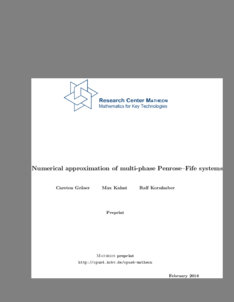Repository: Freie Universität Berlin, Math Department

# Numerical approximation of multi-phase Penrose-Fife systems

Gräser, C. and Kahnt, M. and Kornhuber, R. (2016) Numerical approximation of multi-phase Penrose-Fife systems. Computational Methods in Applied Mathematics, 16 (4). pp. 523-542. ISSN Online: 1609-9389, Print: 1609-4840Preview

2MB

Official URL: http://dx.doi.org/10.1515/cmam-2016-0020

## Abstract

We consider a non-isothermal multi-phase field model. We subsequently discretize implicitly in time and with linear finite elements. The arising algebraic problem is formulated in two variables where one is the multi-phase field, and the other contains the inverse temperature field. We solve this saddle point problem numerically by a non-smooth Schur-Newton approach using truncated non-smooth Newton multigrid methods. An application in grain growth as occurring in liquid phase crystallization of silicon is considered.

Item Type: Article Multi-phase field models, phase transitions, thermodynamic consistency, partial differential inclusion, parabolic variational inequality, finite elements Mathematical and Computer Sciences > Mathematics > Numerical Analysis Department of Mathematics and Computer Science > Institute of Mathematics 1814 Ekaterina Engel 19 Feb 2016 13:01 03 Mar 2017 14:42

Repository Staff Only: item control page